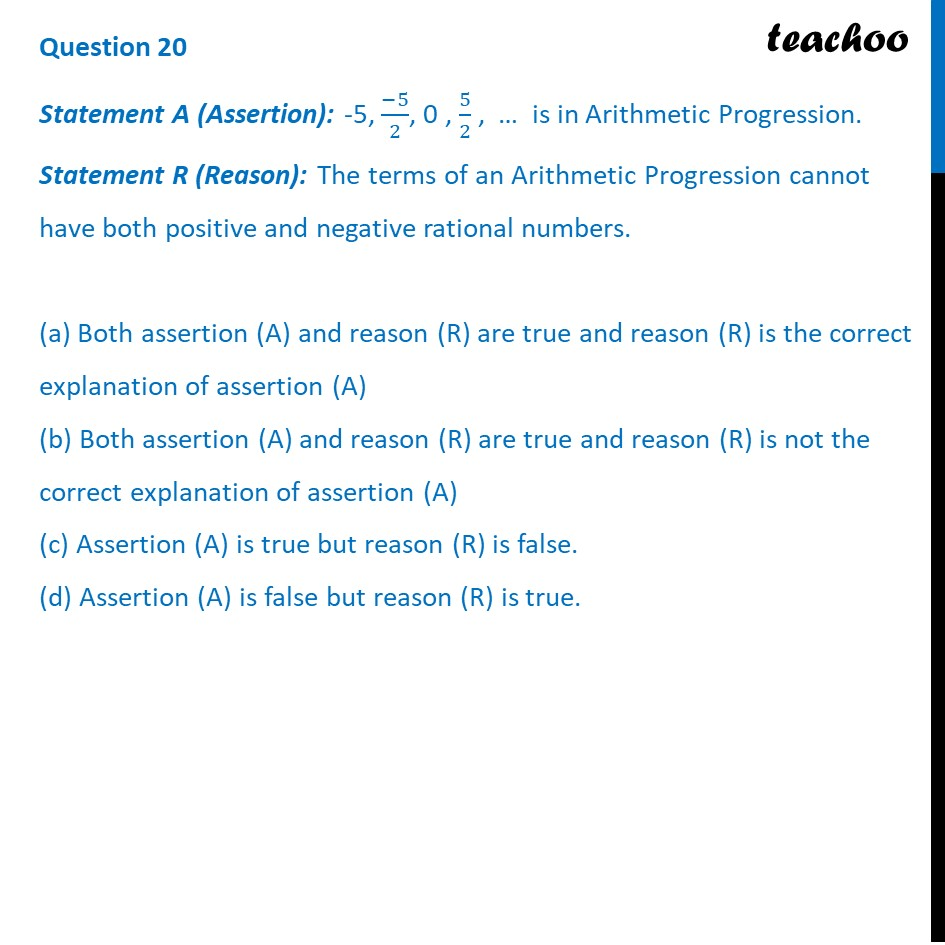CBSE Class 10 Sample Paper for 2024 Boards - Maths Standard

Class 10
Solutions of Sample Papers for Class 10 Boards

## (d) Assertion (A) is false but reason (R) is true.The rest of the post is locked. Join Teachoo Black to see the full post.

### Transcript

We need to check if −5,(−5)/2,0, 5/2,… is an AP or not Now, Difference of 2nd and 1st term = (−5)/2−(−5) = 𝟓/𝟐 And, Difference of 3rd and 2nd term = 0 −((−5)/2) = 𝟓/𝟐 Since difference is same Hence, this sequence forms an AP. Thus, Assertion is true Checking Reason Statement R (Reason): The terms of an Arithmetic Progression cannot have both positive and negative rational numbers. This statement is incorrect as in Assertion we proved that −5,(−5)/2,0, 5/2,… is an AP, And it has Positive rational numbers Negative Rational numbers Thus, Reason is false Thus, Reason is false So, Assertion is true Reasoning is false So, the correct answer is (c)